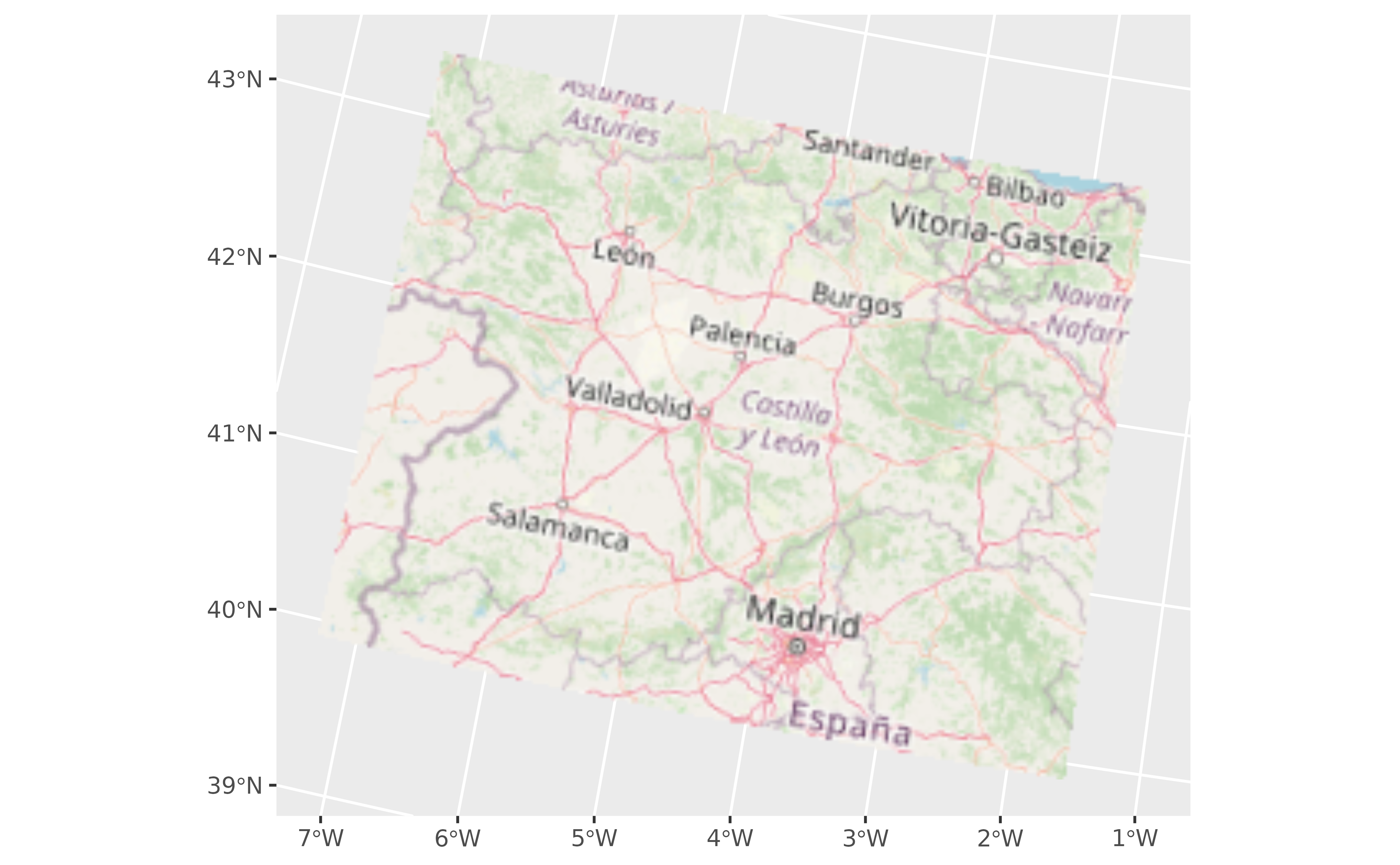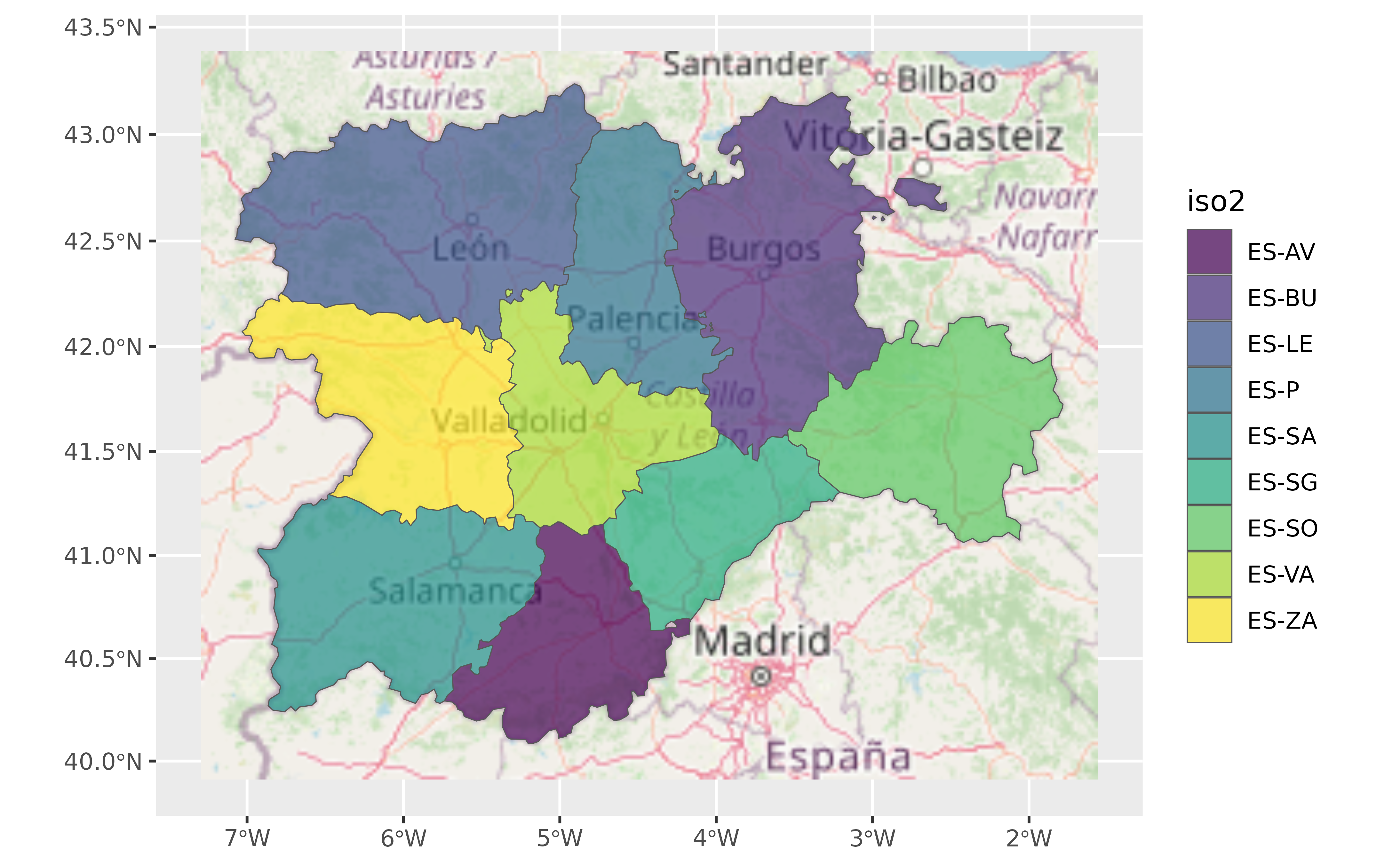This geom is used to visualise SpatRaster objects (see terra::rast()) as RGB images. The layers are combined such that they represent the red, green and blue channel.

For plotting SpatRaster objects by layer values use geom_spatraster().

The underlying implementation is based on ggplot2::geom_raster().

## Usage

geom_spatraster_rgb(
mapping = aes(),
data,
interpolate = TRUE,
r = 1,
g = 2,
b = 3,
alpha = 1,
maxcell = 5e+05,
max_col_value = 255,
...
)

## Source

Based on the layer_spatial() implementation on ggspatial package. Thanks to Dewey Dunnington and ggspatial contributors.

## Arguments

mapping

Ignored.

data

A SpatRaster object.

interpolate

If TRUE interpolate linearly, if FALSE (the default) don't interpolate.

r, g, b

Integer representing the number of layer of data to be considered as the red (r), green (g) and blue (b) channel.

alpha

The alpha transparency, a number in [0,1], see argument alpha in hsv.

maxcell

positive integer. Maximum number of cells to use for the plot.

max_col_value

Number giving the maximum of the color values range. When this is 255 (the default), the result is computed most efficiently. See grDevices::rgb().

...

Other arguments passed on to layer(). These are often aesthetics, used to set an aesthetic to a fixed value, like colour = "red" or size = 3. They may also be parameters to the paired geom/stat.

A ggplot2 layer

## terra equivalent

terra::plotRGB()

## Coords

When the SpatRaster does not present a crs (i.e., terra::crs(rast) == "") the geom does not make any assumption on the scales.

On SpatRaster that have a crs, the geom uses ggplot2::coord_sf() to adjust the scales. That means that also the SpatRaster may be reprojected.

## Aesthetics

No aes() is required. In fact, aes() will be ignored.

ggplot2::geom_raster(), ggplot2::coord_sf(), grDevices::rgb(). You can get also RGB tiles from the maptiles package, see maptiles::get_tiles().

Other ggplot2 utils: autoplot.Spat, fortify.Spat, geom_spat_contour, geom_spatraster(), ggspatvector, stat_spat_coordinates()

## Examples

# \donttest{

# Tile of Castille and Leon (Spain) from OpenStreetMap
file_path <- system.file("extdata/cyl_tile.tif", package = "tidyterra")

library(terra)
tile <- rast(file_path)

library(ggplot2)

ggplot() +
geom_spatraster_rgb(data = tile) +
# You can use coord_sf
coord_sf(crs = 3035)# Combine with sf objects
vect_path <- system.file("extdata/cyl.gpkg", package = "tidyterra")

#> Reading layer cyl' from data source
#>   D:\a\_temp\Library\tidyterra\extdata\cyl.gpkg' using driver GPKG'
#> Simple feature collection with 9 features and 3 fields
#> Geometry type: MULTIPOLYGON
#> Dimension:     XY
#> Bounding box:  xmin: 2892687 ymin: 2017622 xmax: 3341372 ymax: 2361600
#> Projected CRS: ETRS89-extended / LAEA Europe

ggplot(cyl_sf) +
geom_spatraster_rgb(data = tile) +
geom_sf(aes(fill = iso2)) +
coord_sf(crs = 3857) +
scale_fill_viridis_d(alpha = 0.7)# }
`